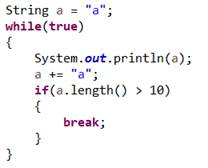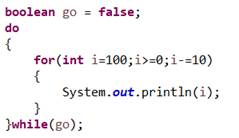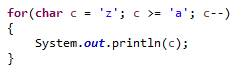Lab 02

Triangle Maker!

Objective:

Write a program that takes in a positive number and draws a horizontal triangle of asterisks (*).  The inputted number represents the size of the triangle, or in other words the number of asterisks at the peak.  It starts out by increasing the number of asterisks at each line until it reaches the size of the triangle and then decreases the number of triangles at each line.

Lab Solution

Requirements:

• Functionality. (80pts)
• No Syntax, Major Run-Time, or Major Logic Errors. (80pts*)
• *Code that cannot be compiled due to syntax errors is nonfunctional code and will receive no points for this entire section.
• *Code that cannot be executed or tested due to major run-time or logic errors is nonfunctional code and will receive no points for this entire section.
• Clear and Easy-To-Use Interface. (10pts)
• Users should easily understand what the program does and how to use it.
• Users should be prompted for input and should be able to enter data easily.
• Users should be presented with output after major functions, operations, or calculations.
• The user must be prompted to enter the size of the triangle.
• All the above must apply for full credit.
• Check for Errors. (10pts)
• The user must enter a non-zero, positive, whole number that corresponds to the size of the triangle.
• If the user enters anything else, then the program should inform the user of the error then immediately terminate.
• All the above must apply for full credit.
• Drawing the Triangle. (60pts)
• The program must draw the triangle in the console using asterisks (“*”).
• The triangle drawn must start with a single asterisk, then increase the number of asterisks line-by-line until the size is reached, and finally decrease the number of asterisks line-by-line until a single asterisk remains.
• An example would be if the user inputs “4”, then the triangle below would be drawn,

*

**

***

****

***

**

*

• All the above must apply for full credit.
• Coding Style. (10pts)
• Meaningful identifiers for data and methods.
• Proper indentation that clearly identifies statements within the body of a class, a method, a branching statement, a loop statement, etc.
• All the above must apply for full credit.
• Your name in the file. (5pts)
• At least 5 meaningful comments in addition to your name. These must describe the function of the code it is near. (5pts)

Example Dialog:

Welcome to Triangle Maker!  Enter the size of the triangle.

3

*

**

***

**

*

DONE!

Solution Tests:

1. Is your name written as a comment in all source files?
2. Does the solution compile (no syntax errors)?
3. Enter the value 3. Does the output match the triangle below?

*

**

***

**

*

1. Enter the value 5. Does the output match the triangle below?

*

**

***

****

*****

****

***

**

*

1. Enter the value -1. Does the program stop immediately?

Lab Report

1. Create a section named “Problem” and describe this lab’s problem in your own words. (10pts).
2. Create a section named “Solution Description” and describe how the code solves the problem in your own words. (10pts).
3. Create a section named “Problems Encountered” and describe the various syntax, run-time, and logic errors that were encountered while implementing the solution. (10pts).
4. Draw a flow chart for the solution of this problem.
5. When specifically is it more appropriate to use a for-loop rather than a while loop?
6. Turn the following for-loop into an equivalent while-loop

for(int i=100;i>0;i-=10)

{

System.out.println(i);

}

1. Given the following code snippet, are there any kind of errors? If so, name the types, where, and how this code can be fixed. Otherwise, what does this code print to the console?1. Given the following code snippet, are there any kind of errors? If so, name the types, where, and how this code can be fixed. Otherwise, what does this code print to the console?1. Given the following code snippet, are there any kind of errors? If so, name the types, where, and how this code can be fixed. Otherwise, what does this code print to the console?1. The following code snippet is suppose to print out a 10 by 10 box with numbers from 0 to 99, but it does not seem to work. What error(s) are causing the problem and how can they be fixed?Finally:

Upload the source code (.JAVA File Extension) and written lab report (.DOC, .DOCX, or .PDF file extension) to the CSCE Dropbox.